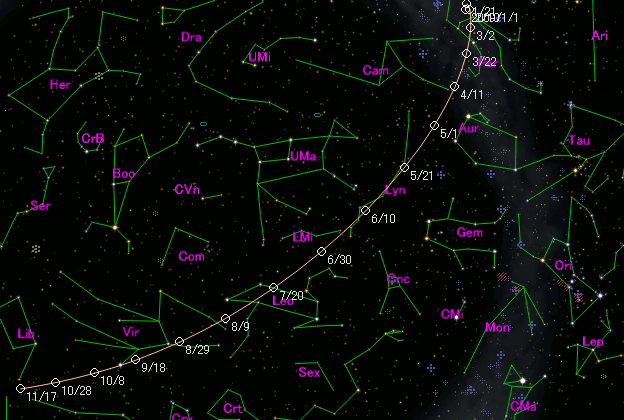# \$B%.%V%9WB@1(B

C/2009 K4 ( Gibbs )###\$B%W%m%U%#!<%k(B

 \$BH/8+F|(B 2009\$BG/(B5\$B7n(B27\$BF|(B \$BH/8+8wEY(B 17.0\$BEy(B \$BH/8+ A. R. Gibbs (Catalina)

###\$B###\$B50F;MWAG(B

```Epoch 2009 June 18.0 TT = JDT 2455000.5
T 2009 June 19.37894 TT                                 MPC
q   1.5490829            (2000.0)            P               Q
z  +0.0235930      Peri.  127.46772     -0.85313329     -0.43593790
+/-0.0000000      Node    30.12010     +0.05670379     -0.62353734
e   0.9634526      Incl.   34.82458     +0.51860223     -0.64896790
```

###\$B@1?^(B###\$B8wEYJQ2=(B

```        m1 = 11.5 + 5 log\$B&\$(B + 10.0 log r
```##### \$B50F;MWAG\$O(BM.P.E.C. 2009-P12\$B\$K7G:\\$5\$l\$?\$b\$N\$G\$9!#(B \$B@1?^\$O%9%F%i%J%S%2!<%?(B Ver.8 (\$B%"%9%H%m%"!<%D(B) \$B\$G:n@.\$7\$?\$b\$N\$G\$9!#(B \$B8wEY%0%i%U\$O(BComet for Windows\$B\$G:n@.\$7\$?\$b\$N\$G\$9!#(B